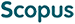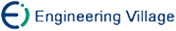丁祥武, 郭涛, 王梅, 金冉. 一种大规模分类数据聚类算法及其并行实现[J]. 计算机研究与发展, 2016, 53(5): 1063-1071.
 引用本文: 丁祥武, 郭涛, 王梅, 金冉. 一种大规模分类数据聚类算法及其并行实现[J]. 计算机研究与发展, 2016, 53(5): 1063-1071.Ding Xiangwu, Guo Tao, Wang Mei, Jin Ran. A Clustering Algorithm for Large-Scale Categorical Data and Its Parallel Implementation[J]. Journal of Computer Research and Development, 2016, 53(5): 1063-1071.
 Citation: Ding Xiangwu, Guo Tao, Wang Mei, Jin Ran. A Clustering Algorithm for Large-Scale Categorical Data and Its Parallel Implementation[J]. Journal of Computer Research and Development, 2016, 53(5): 1063-1071.## A Clustering Algorithm for Large-Scale Categorical Data and Its Parallel Implementation

Abstract: CLOPE algorithm has achieved good results in clustering large, sparse categorical datasets with high dimensions. However, it is hard to stably find the global optimal clusters since the data order can affect the result of clustering. To deal with this problem, this paper proposes p-CLOPE algorithm iteratively dividing input data into multiply equal parts and then clustering their different permutations. In each iteration of p-CLOPE algorithm, the input dataset is split into p parts and they are permuted into p! datasets with different part orders, then each dataset is clustered and the optimal clustering is chosen according to the profit as the input of next iterations. In order to handle time complexity of the process, a result reusing strategy is put forward that can improve the speed of clustering, further. Finaly, a distributed solution is put forward that implements p-CLOPE on Hadoop platform and a clustering tool is developed which has been released to the open source community. Experiments show that p-CLOPE can achieve better results than CLOPE. For the Mushroom dataset, when CLOPE achieves optimal results, p-CLOPE can achieve 357% higher profit value than CLOPE. When dealing with big data, parallel p-CLOPE greatly shortens the computing time compared with serial p-CLOPE, and it achieves nearly p! speedup when there is enough computing resource./下载:  全尺寸图片 幻灯片
• 分享
• 用微信扫码二维码

分享至好友和朋友圈